# DCN椎体神经元的Hopf分岔控制Hopf Bifurcation Control of DCN Pyramidal Neurons

• 全文下载: PDF(835KB)    PP.133-141   DOI: 10.12677/AAM.2020.92016
• 下载量: 40  浏览量: 77

>Pyramidal cell in the dorsal cochlear nucleus (DCN) is one of the first neurons in the central au-ditory system to process acoustic signals, which plays an important role in the processing and integration of acoustic information. The KM model based on voltage dependent ion channel var-iables can be established by the in vitro experimental data of DCN pyramidal neurons. We use hf as a bifurcation parameter to analyze the fast and slow dynamics of DCN pyramidal neurons. Then, a dynamic state-feedback control law incorporating a washout filter is used to control the Hopf bifurcation position of KM model, and our control task is realized.

1. 引言

2. Kanold-Manis DCN椎体神经元模型(KM模型)

$\begin{array}{l}Cm\stackrel{˙}{V}=I-{\stackrel{¯}{g}}_{Na}{m}_{Na}^{2}{h}_{Na}\left(V-{V}_{Na}\right)-\left({\stackrel{¯}{g}}_{Kni}{n}_{Kni}^{2}+{\stackrel{¯}{g}}_{Kif}{m}_{f}^{4}{h}_{f}+{\stackrel{¯}{g}}_{Kis}{m}_{s}^{4}{h}_{s}\right)\left(V-{V}_{K}\right)-{g}_{L}\left(V-{V}_{L}\right)-{\stackrel{¯}{g}}_{h}{m}_{h}^{4}{n}_{h}\left(V-{V}_{h}\right)\\ \stackrel{˙}{x}=\frac{{x}_{\infty }\left(V\right)-x}{{\tau }_{x}\left(V\right)}\\ x={h}_{Na},{m}_{Na},{n}_{Kni},{m}_{h},{n}_{h},{m}_{f},{h}_{f},{m}_{s},{h}_{s}\end{array}$

DCN椎体神经元模型的具体通道开关概率参数如下： ${\theta }_{mNa}=-38$${\theta }_{hNa}=-43$${\theta }_{nKni}=-40$${\theta }_{mh}=-68.9$${\theta }_{nh}=-68.9$${\theta }_{mf}=-53$${\theta }_{hf}=-38$${\theta }_{ms}=-40.9$${\theta }_{hs}=-38.4$${k}_{mNa}=-3$${k}_{nKni}=-3$${k}_{hna}=3$${k}_{mh}=6.5$${k}_{nh}=6.5$${k}_{mf}=-25.8$${k}_{hf}=6.5$${k}_{ms}=-23.7$${k}_{hs}=9$ 。膜电容 ${C}_{m}=12.5\text{\hspace{0.17em}}\text{pF}$ 。最大通道电导常数(单位：nS)为： ${\stackrel{¯}{g}}_{Na}=350$${\stackrel{¯}{g}}_{Kni}=80$${\stackrel{¯}{g}}_{h}=3$${\stackrel{¯}{g}}_{Kif}=150$${\stackrel{¯}{g}}_{Kis}=40$${g}_{l}=2.8$ 。各离子电流的可逆电位(单位：mV)为： ${V}_{K}=-81.5$${V}_{Na}=50$${V}_{L}=-57.7$${V}_{h}=-43$

3. KM模型的分岔分析

3.1. 快慢动力学分析

$\left\{\begin{array}{l}\stackrel{˙}{x}=f\left(x,y\right)\text{\hspace{0.17em}}\text{ }\text{\hspace{0.17em}}\text{\hspace{0.17em}}\left(快子系统\right)\\ \stackrel{˙}{y}=\epsilon g\left(x,y\right)\text{ }\text{\hspace{0.17em}}\left(慢子系统\right)\end{array}$

$x\in {R}^{m},\text{\hspace{0.17em}}y\in {R}^{k},\text{\hspace{0.17em}}\epsilon \ll 1$ 向量 $x\in {R}^{m}$ 表示对应于放电形式的快变量。它包含膜电位V以及快电流的激活或非激活的门控变量，等等。向量 $y\in {R}^{k}$ 表示调制快放电的相关的慢变量，比如，慢钾电流的门控变量、内钙离子浓度等。小参数表示快慢时间尺度的比率，也即放电与调制的时间比率。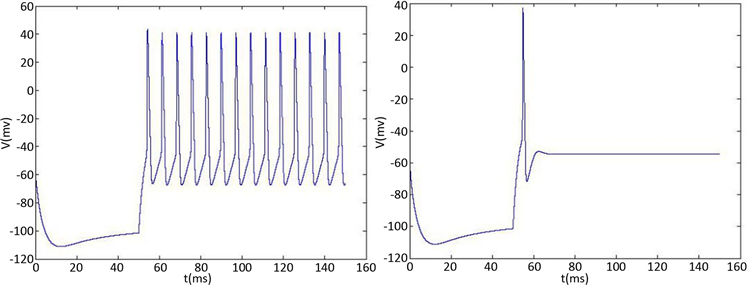(a1) (a2)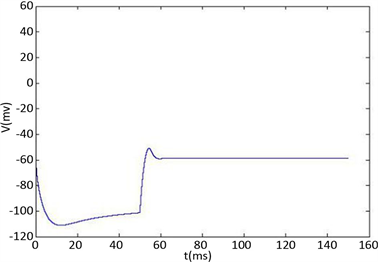(a3)

Figure 1. Time course of the membrane potential V stimulated by square wave current stimulated by hyperpolarization and depolarization of the KM model at different ${h}_{f}$ . (a1)~(a3) The current response of the KM model. The selected depolarization current is 140 pA, and the 50 ms hyperpolarization current is −200 pA. The change of ${h}_{f}$ is 1, 0.5, 0.05 in turn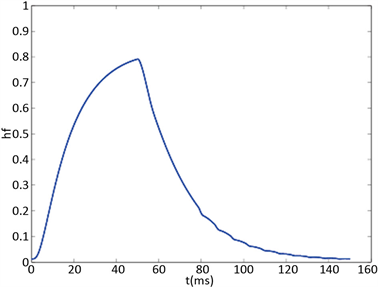Figure 2. KM model (a) Time history of fast potassium current shutdown probability variable ${h}_{f}$ . In the hyperpolarized state, ${h}_{f}$ gradually increases, and in the depolarized state, ${h}_{f}$ gradually decreases. The selected depolarization current is 140 pA, and the 50 ms hyperpolarization current is −200 pA

3.2. KM模型的快慢动力学分岔分析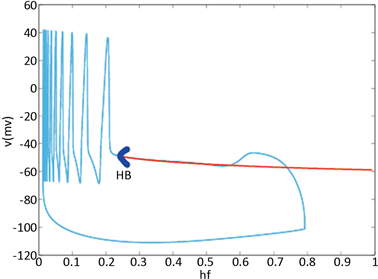Figure 3. Analysis of fast and slow dynamic bifurcation of KM model. The slow variable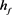is a parameter, the red line represents the stability point of the fast subsystem, and the blue line represents the maximum and minimum values of the limit cycle. The blue-grey line indicates the trajectory of the system under the stimulation of hyperpolarized current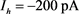and depolarized current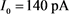4. KM模型的Hopf分岔控制

4.1. Hopf分岔出现的条件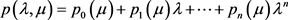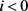或者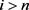时，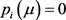。我们再令1)2)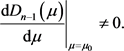4.2. 冲洗滤波器的动态状态反馈控制律4.3. 受控制的KM模型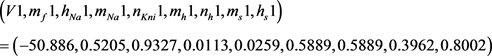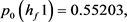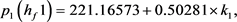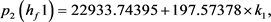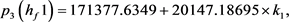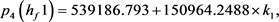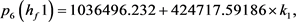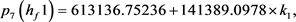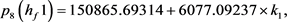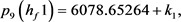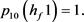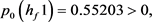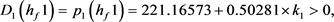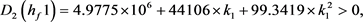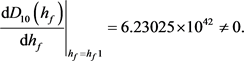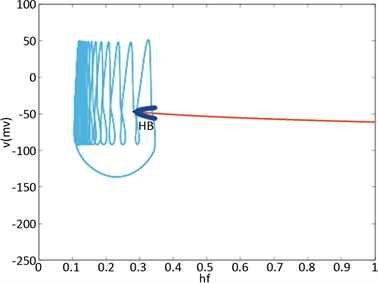Figure 4. KM model after control. The slow variableis a parameter, the red line represents the stability point of the fast subsystem, and the blue line represents the maximum and minimum values of the limit cycle. The blue-grey line indicates the trajectory of the system under the stimulation of hyperpolarized current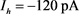and depolarized current5. 结论

  Luo, M., Wu, Y.J. and Peng, J.H. (2009) Washout Filter Aided Mean Field Feedback Desynchronization in an En-semble of Globally Coupled Neural Oscillators. Biological Cybernetics, 101, 241-246. https://doi.org/10.1007/s00422-009-0334-5  Crawford, J.D. (1991) Introduction to Bifurcation Theory. Review of Modern Physics, 63, 991-1038. https://doi.org/10.1103/RevModPhys.63.991  Huang, C.L., Sun, W., Zheng, Z.G., et al. (2016) Hopf Bi-furcation Control of the M-L Neuron Model with Type I. Nonlinear Dynamics, 87, 755-766.  Xie, Y., Aihara, K. and Kang, Y.M. (2008) Change in Types of Neuronal Excitability via Bifurcation Control. Physical Review E, 77, Article ID: 021917. https://doi.org/10.1103/PhysRevE.77.021917  Nguyen, L.H., Hong, K.S. and Park, S. (2012) Bifurcation Control of the Morris-Lecar Neuron Model via a Dynamic State-Feedback Control. Biological Cybernetics, 106, 587-594. https://doi.org/10.1007/s00422-012-0508-4  Kanold Patrick, O. and Manis Paul, B. (2001) A Physiologically Based Model of Discharge Pattern Regulation by Transient K+ Currents in Cochlear Nucleus Pyramidal Cells. Journal of Neurophysiology, 85, 523-538. https://doi.org/10.1152/jn.2001.85.2.523  Augustine, G.J. (1990) Regulation of Transmitter Release at the Squid Giant Synapse by Presynaptic Delayed Rectifier Potassium Current. The Journal of Physiology, 431, 343-364. https://doi.org/10.1113/jphysiol.1990.sp018333  Liu, W.M. (1994) Criterion of Hopf Bifurcations with-out Using Eigenvalues. Journal of Mathematical Analysis and Applications, 182, 250-256. https://doi.org/10.1006/jmaa.1994.1079